17th of September, 2008

1. The potential must be the same everywhere inside the sphere. This condition is satisfied if the charge density σfree + σbound is constant on the surface of the sphere and 0 everywhere else. It can easily be seen that this configuration is a valid solution because bound charges can be induced only on the surface of a homogeneous and linear dielectric if the normal component of the electric field is non-zero there.

It can be proven that this is the only solution using an argument similar to that found in Griffiths’ book (page 118, second uniqueness theorem), but using the displacement vector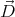instead of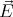.

If the sphere is pushed “deeper” into the dielectric (fig. 2a), the potential cannot stay unchanged because the electric field will no longer be parallel to the surface of the dielectric and bound charges will be induced. In the case illustrated on fig. 2b, the potential will not change.

If the total charge of the sphere is constant, the charges will redistribute on the surface of the sphere in such a way that the electric field can stay spherically symmetric. On the lower hemisphere the bound charges will reduce the total (bound + free) surface charge density by a factor of ε. Thus, if the total free charge is Qfree, and the total charge is Q, Q∕2 + εQ∕2 = Qfree Q = 2Qfree(1 + ε). The potential will be reduced by a factor of 2(1 + ε) everywhere in space.

2. See Griffiths, page 194.
3. Carving a cavity inside a polarized dielectric is equivalent to superposing a region of equal but opposite polarization. Since the dielectric is uniformly polarized, all we need to do is find the electric field inside a uniformly polarized spherical, needle- and wafer-shaped region, and superpose this field with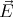0.

The polarization of the dielectric is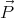0 = ε0χ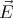0 where χ = εr -1 is the susceptibility of the material. We shall need to consider regions with uniform polarization -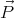0.

1. Spherical region

What is the electric field inside a uniformly polarized sphere, of polarization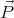?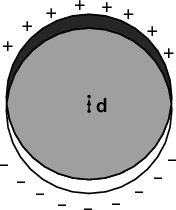A uniformly polarized sphere can be imagined as two superposed uniformly but oppositely charged spheres, slightly displaced by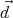. The electric field inside a uniformly charged sphere with charge density ρ, at position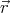relative to the sphere’s centre is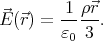The electric field inside the superposed spheres is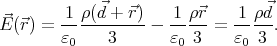The polarization vector can be thought of as a density of electric dipole moments, so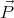= ρ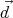, and the electric field inside the polarized region isThe electric field in a spherical cavity is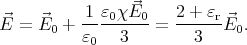2. Needle-shaped region Let us denote the tiny base surface of the needle with A. Then the bound charges induced by the polarization will be two point like charges of magnitude AP at the tips of the needle.

Thus the electric field in the middle of a needle of length L is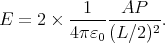The electric field in a cavity is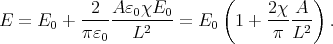3. Wafer-shaped region A polarized flat region is just like the capacitor: two parallel sheets of bound charges, with surface charge density σ = P. The electric field inside this region is just E = σ∕ε0 = P∕ε0.

Thus the electric field in a thin wafer shaped cavity is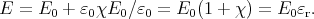4. What is the dipole moment of a dielectric sphere placed in a homogeneous electric field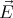0? As shown in the previous exercise, the electric field created by a polarized sphere is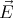inside =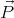(3ε0). The total electric field in the sphere is=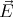0 -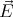inside and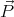= ε0χ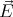= ε0(εr - 1)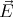, so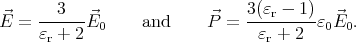The dipole moment of a sphere of volume V and polarization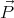is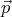= V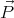, so the polarizability of a sphere is α = 3V ε0.

Let us assume that that all the droplets have volume V and there are N droplets per unit volume: NV = β.

By definition, if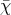is the susceptibility of the emulsion,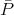its polarization, and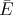the electric field inside, then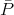=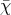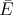. If the density of the droplets is low (β 1), we can consider the field polarizing a droplet to be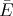, so p = αand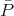= Np =. In this case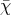= and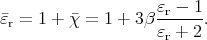However,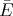is the average field in the emulsion, and a precise treatment of the problem must consider the local field polarizing a droplet (see problem 4.38 in Griffiths). Because of the distorting effect of the neighbouring droplets, this local field will be different from the average field. To approximate this local field, imagine that we remove the droplet, creating a spherical cavity in the emulsion. The electric field in this cavity will be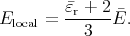Using this formula,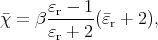and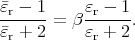5. The vz component of the velocity vector is constant, so we only need to consider particles moving in the xy plane.
1. The radius of curvature of the particle’s trajectory is R = mv∕qB. It is easy to see that the particle will drift along the ŷ direction. On the figure, the magnetic field increases and the radius of curvature decreases with x.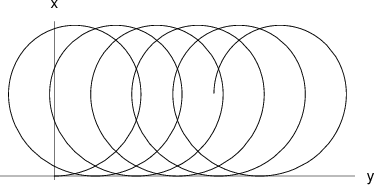2. The drift velocity is the particle’s velocity vector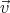averaged over a complete cycle in the periodic trajectory: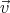D =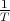0T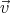dt =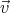. In a homogeneous magnetic field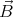0, the average of the velocity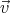0 is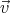0= 0. If the magnetic field is perturbed with a small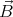, the change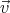(t) =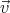(t) -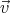0(t) in the velocity will also be small. Note that this is true only because in this problem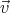(t) is periodic.

The motion of the particle is governed by the equation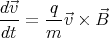Let us take the average of both sides of this equation over a complete cycle: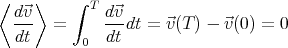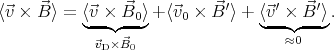Thus,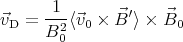For calculating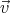0 ×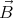′⟩, we again approximate the actual trajectory with the non-perturbed one: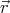= R(cos ωt, sin ωt, 0)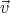0 =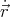= v(- sin ωt, cos ωt, 0)
 (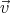0 ×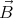′)x = (v0)ykx = kRv cos 2ωt (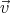0 ×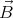′)y = -(v0)xkx = kRv sin ωt cos ωt
Integrating these expressions over (0,T) we get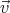0 ×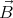′⟩ = Rv0 k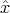2. Defining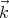= k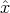, the drift velocity is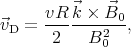where R = mv∕qB0.

Back to the main page